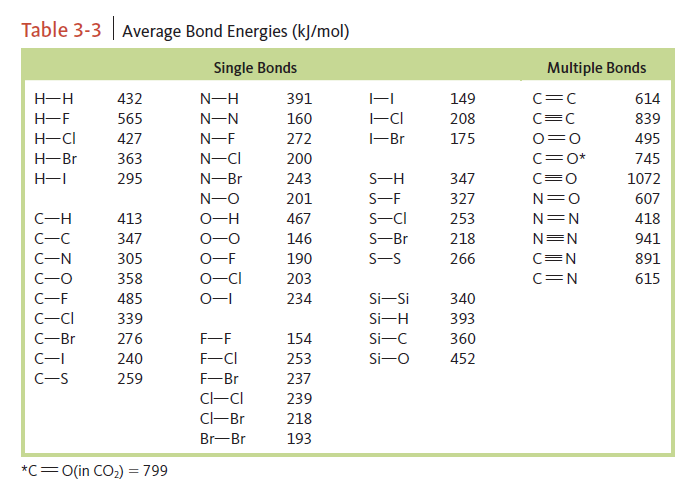# Problem: Consider the following reaction:H2O(g) + Cl 2O(g) → 2HOCl(g)     K 298 = 0.090For Cl2O(g),       ΔG°f = 97.9 kJ/mol       ΔH°f = 80.3 kJ/mol           S° = 266.1 J/K • molb. Use bond energy values from the following table to estimate ΔH° for the reaction.

###### FREE Expert Solution

To calculate the ΔH°rxn using bond energies, we’re going to use the following equation:

$\overline{){\mathbf{∆}}{\mathbf{H}}{{\mathbf{°}}}_{{\mathbf{rxn}}}{\mathbf{=}}{\mathbf{∆}}{\mathbf{H}}{{\mathbf{°}}}_{{\mathbf{products}}}{\mathbf{-}}{\mathbf{∆}}{\mathbf{H}}{{\mathbf{°}}}_{{\mathbf{reactants}}}}$

Balanced Reaction:  H2O(g) + Cl 2O(g) → 2HOCl(g)

*always make sure that the given reaction is balanced

79% (458 ratings)###### Problem Details

Consider the following reaction:

H2O(g) + Cl 2O(g) → 2HOCl(g)     K 298 = 0.090

For Cl2O(g),
ΔG°f = 97.9 kJ/mol
ΔH°f = 80.3 kJ/mol
S° = 266.1 J/K • mol

b. Use bond energy values from the following table to estimate ΔH° for the reaction.